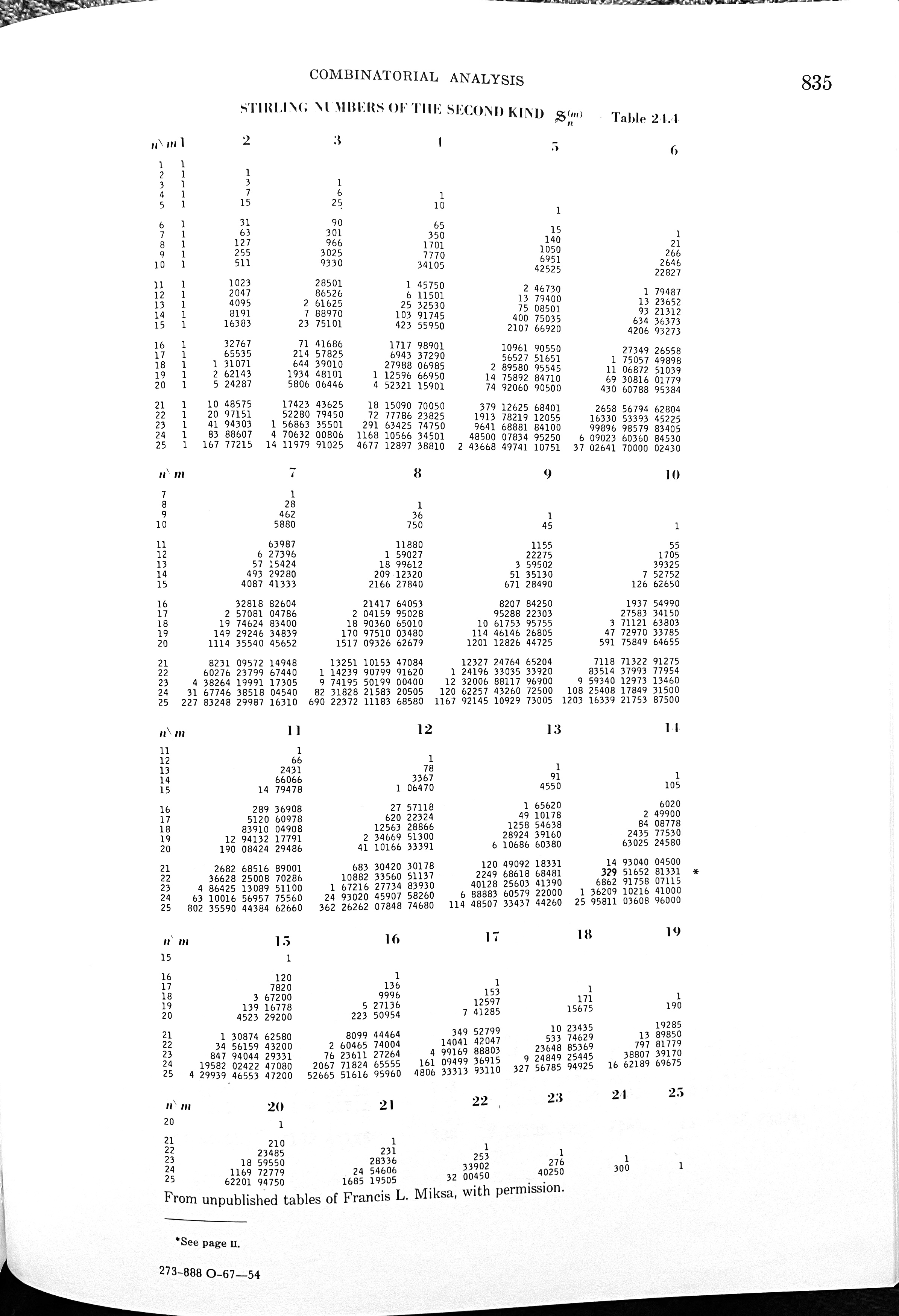# Trump combinatorics

This afternoon, there will be a hearing in the Georgia state court case against Donald Trump and his 18 co-defendants. Judge Scott McAfee ordered the hearing yesterday and asked DA Fanni Willis’s office to make

[a] good-faith estimate of the time reasonably anticipated to present the State’s case during a joint trial of all 19 co-defendants, and alternatively any divisions thereof, including the number of witnesses likely to be called and the number and size of exhibits likely to be introduced.

Emphasis added because that’s the point of this post.

Taken at face value, McAfee is asking Willis to make these estimates for a single trial, 19 separate trials, and every possibility in between. Since this is an impossible task because of the monstrous number of trial combinations, we don’t take him at face value. But what if we did? How many different ways could this case be split into separate trials?

Obviously, there’s just one way to have a single trial of all the defendants and just one way to have 19 trials, each with an individual defendant. Let’s consider the next arrangement on the complication scale: 18 trials. This would mean one trial with 2 defendants and 17 trials with individual defendants. The key to working out this figure is determine the number of ways we can pair 2 defendants from the 19. For that we need the binomial coefficient:

$\binom{19}{2} = \frac{19!}{2! \, 17!} = 171$

The next most complicated arrangement is two trials. For this, we need to consider the nine ways to split up the defendants and the number of combinations associated with each of those splits.

Split of defendants Formula Count
1 and 18 $$\dbinom{19}{2}$$ 19
2 and 17 $$\dbinom{19}{2}$$ 171
3 and 16 $$\dbinom{19}{3}$$ 969
4 and 15 $$\dbinom{19}{4}$$ 3,876
5 and 14 $$\dbinom{19}{5}$$ 11,628
6 and 13 $$\dbinom{19}{6}$$ 27,132
7 and 12 $$\dbinom{19}{7}$$ 50,388
8 and 11 $$\dbinom{19}{8}$$ 75,582
9 and 10 $$\dbinom{19}{9}$$ 92,378
Total 262,143

Two of those numbers should be familiar.

At this point, I think it’s time to give up on the binomial coefficient. There may be a way to use it to work out the number of ways to have three trials, four trials, and so on up to 17 trials, but I don’t want try it. More powerful tools are available, and we should take advantage of them.

The Stirling numbers of the second kind are what we need. As the MathWorld article says, they are

[t]he number of ways of partitioning a set of n elements into m nonempty sets…

The key words here are partitioning and nonempty. When we partition a set into subsets, the subsets do not intersect with each other and their union is the original set. Translated to our problem, that means each defendant is in one and only one trial. And the subsets are nonempty because we can’t have a trial with no defendant.

The Stirling numbers of the second kind are in the OEIS, but the list on that page doesn’t go up high enough. The tables in Abramowitz & Stegun do,but there’s no way I can enter the numbers for $$n = 19$$ without making several typos. So let’s fire up Mathematica and use its StirlingS2 function

Table[{n, StirlingS2[19, n]}, {n, 1, 19}]


yields

{{1, 1},
{2, 262143},
{3, 193448101},
{4, 11259666950},
{5, 147589284710},
{6, 693081601779},
{7, 1492924634839},
{8, 1709751003480},
{9, 1144614626805},
{10, 477297033785},
{11, 129413217791},
{12, 23466951300},
{13, 2892439160},
{14, 243577530},
{15, 13916778},
{16, 527136},
{17, 12597},
{18, 171},
{19, 1}}


where the first number in each line is the number of trials and the second is the number of ways to arrange the defendants in that many trials. We see that the values for 1, 2, 18, and 19 trials match what we came up with earlier, and now we have all the others, too. If your eyes are good, you can compare the numbers in the middle to the A&S table.

To get the total, we run

Total[Table[StirlingS2[19, n], {n, 1, 19}]]


to get 5,832,742,205,057, or over 5.8 trillion possibilities. I suggest we call this the McAfee number.

Update 7 Sep 2023 1:53 PM
Reader Rick Kaye, who has probably forgotten more combinatorics than I’ll ever know, emailed me to point out that the number of ways to partition a set into nonempty subsets is the Bell Number. In Mathematica, it’s calculated through the BellB function, so

BellB


returns 5,832,742,205,057, the same value I got by summing the Stirling numbers of the second kind. You can check this via

BellB == Total[Table[StirlingS2[19, n], {n, 1, 19}]]


which returns True. Also, the Bell Numbers are sequence A000110 in the OEIS, where you can look up the value directly. Thanks, Rick!